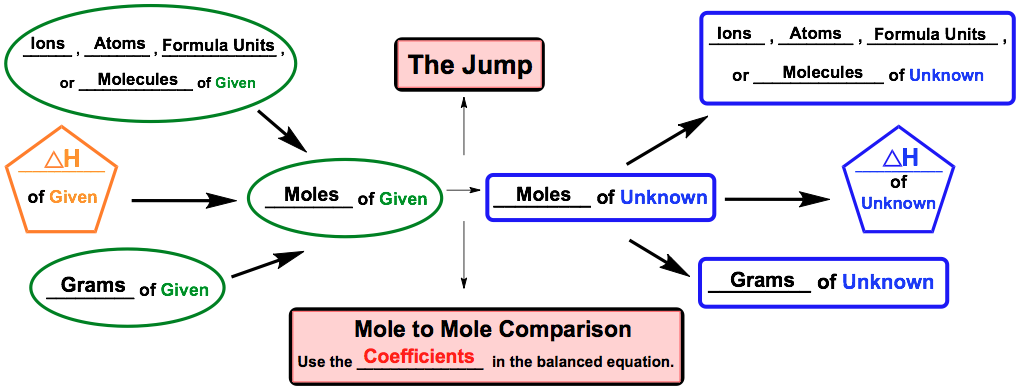Clutch Prep is now a part of Pearson
Ch.6 - Thermochemistry WorksheetSee all chapters

# Thermochemical Equations

See all sections
Sections
Nature of Energy
Kinetic & Potential Energy
First Law of Thermodynamics
Internal Energy
Endothermic & Exothermic Reactions
Heat Capacity
Constant-Pressure Calorimetry
Constant-Volume Calorimetry
Thermal Equilibrium
Thermochemical Equations
Formation Equations
Enthalpy of Formation
Hess's Law
Enthalpy (IGNORE)

Thermochemical Equations involve a balanced chemical equation with a given enthalpy value.

###### Thermochemical Equations

Concept #1: Thermochemical Equations

Concept #2: Thermochemical Stoichiometric Chart

A modified version of the stoichiometric chart can used for thermochemical equations.Example #1: Consider the following thermochemical reaction:

How many grams of MgO are produced during an enthalpy change of –375 kJ?

Practice: Nitromethane (CH3NO2), sometimes used as a fuel for drag racing, burns according to the following reaction:

4 CH3NO2 (l) + 7 O2 (g) → 4 CO2 (g) + 6 H2O (g) + 4 NO2 (g)      ∆Hº = – 2441.6 kJ

How much heat is released by burning 125.0 g of nitromethane (MW: 61.044 g/mol)?

Practice: Consider the following reaction:

2 C6H6 (l) + 15 O2 (g) → 12 CO2 (g) + 6 H2­O (g)   ∆Hº = – 6278 kJ

What volume of benzene (C6H6, d = 0.880 g/mL, molar mass = 78.11 g/mol) is necessary to evolve 5.19 x 109 kJ of heat?

Practice: The creation of liquid methanol is accomplished by the hydrogenation of carbon monoxide:

CO (g) + 2 H2 (g) → CH3OH (l)       ∆Hº = – 128.1 kJ

How much heat (in kJ) is released when 125.0 g CO reacts with 2.32 x 102 g H2?